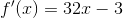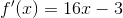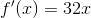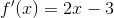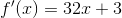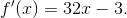# Calculus 2 : First and Second Derivatives of Functions

## Example Questions

### Example Question #271 : Derivative Review

Find the first derivative of the following function: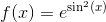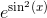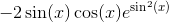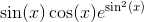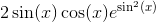Explanation:

The first derivative of the function is equal to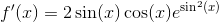and was found using the following rules: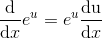,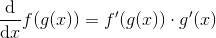,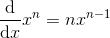,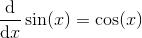### Example Question #272 : Derivative Review

Find the first derivative of the function: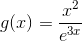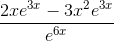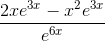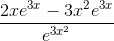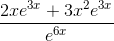Explanation:

The first derivative of the function is equal to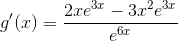and was found using the following rules: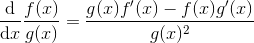,,### Example Question #273 : Derivative Review

Find the first derivative of the function: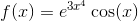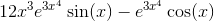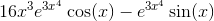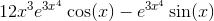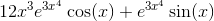Explanation:

The derivative of the function is equal to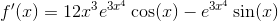and was found using the following rules: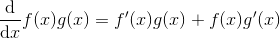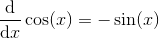### Example Question #274 : Derivative Review

Find the derivative of the function: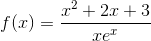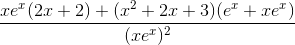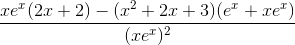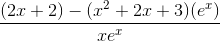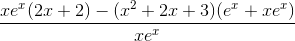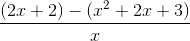Explanation:

The derivative of the function is equal to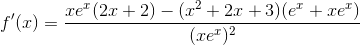and was found using the following rules: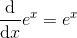### Example Question #275 : Derivative Review

What is the derivative of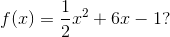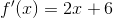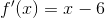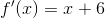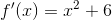Explanation:

To take the derivative, remember to multiply the exponent by the coefficient in front of the x term and then subtract one from the exponent. Therefore, your answer should be: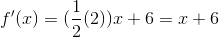.

### Example Question #276 : Derivative Review

What is the acceleration function if the position function is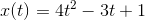?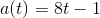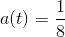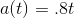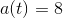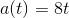Explanation:

Recall that the acceleration function is the second derivative of the position function. So, the first step is taking the first derivative. Remember to multiply the exponent by the coefficient in front of the x term and then subtract the exponent by 1. The first derivative is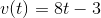. Then, take the derivative of the velocity function to get the acceleration function, which is.

### Example Question #277 : Derivative Review

What is the derivative of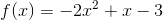?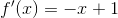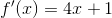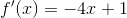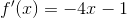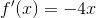Explanation:

Remember that when taking the derivative, multiply the exponent by the coefficient in front of the x and then subtract one from the exponent. Therefore, your answer is:.

### Example Question #278 : Derivative Review

Find the derivative of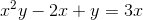.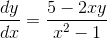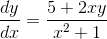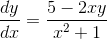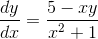Explanation:

To find the derivative, remember to use implicit differentiation.

To find the derivative take the derivative of each term.

In this particular case the power rule,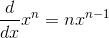and the product rule,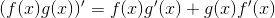will be applied to solve.

Your first step should look like this: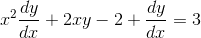.

Then, solve for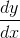.

The next step should look like: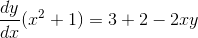..

### Example Question #279 : Derivative Review

What is the derivative of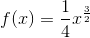?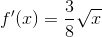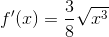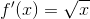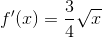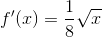Explanation:

To find the derivative, multiply the exponent by the coefficient in front of the x and then subtract 1 from the exponent.

Therefore, the first step is: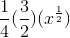..

### Example Question #280 : Derivative Review

What is the derivative of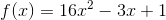?